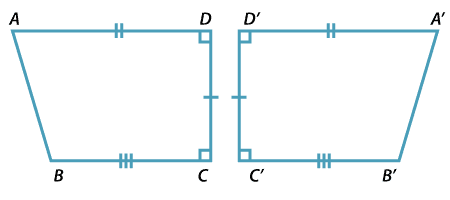When two figures are congruent, we can match every part of one figure with the corresponding part of the other figure. For example, the two figures below are congruent.

Some of the matchings of points with points, intervals with intervals, and angles with angles are:

$$A\leftrightarrow A'$$ $$AB\leftrightarrow A'B'$$ $$\angle BAD\leftrightarrow \angle B'A'D'$$

Matching intervals have the same length, and matching angles have the same size.  $$ABCD$$ and $$A^\prime B^\prime C^\prime D^\prime$$ have the same area.Quadrilateral $$A^\prime B^\prime C^\prime D^\prime$$ is obtained from quadrilateral $$ABCD$$ by a reflection. Notice what happens when you start to go around each quadrilateral in a clockwise direction starting at $$A$$ in the first and $$A'$$ in the second.